"Spectra of discrete two-dimensional periodic Schrödinger operators with small potentials"
Mark Embree and Jake Fillman
Preprint: arXiv:1701.00863 [math.SP] (January 2017)

### What it does

We are interested in the spectral properties of quasicrystals, as modeled by discrete Schrödinger operators with aperiodic potentials. Such systems can be approximated by operators with periodic potentials. In one dimension, much is understood: Adding a small aperiodic potential to the Laplacian can open gaps throughout the spectrum, even giving a spectrum that is a Cantor set. More precisely, if $\Delta$ represents the Laplacian on a regular one-dimensional lattice, and $V$ comes from a broad class of aperiodic potentials, the spectrum of $\Delta + \lambda V$ is $[-2,2]$ when $\lambda=0$ and is a Cantor set for all $\lambda>0$.

We investigate whether the same phenomenon holds in higher dimensions. In particular, we study discrete Schrödinger operators on a (doubly infinite) 2d square lattice, with potentials $V$ that are periodic in both directions, having periods $p$ and $q$. In this case, the spectrum of the Laplacian $\Delta$ is $[-4,4]$. Extending work by Helge Krüger, we demonstrate that for suffiently small $\lambda$, the spectrum of $\Delta+\lambda V$ is one interval (if $p$ or $q$ is odd) or at most two intervals with a gap opening at $E=0$ (if both $p$ and $q$ are even).

### Why it matters

Periodic potentials (with large $p$ and $q$) arise as approximations of aperiodic potentials. By better understanding the periodic case, and how much it differs from the one-dimensional analog, we gain insight into possible spectral structures for aperiodic operators with small potentials. For example, our results immediately give some insight into the spectra of limit periodic operators, via Krüger's Theorem 7.1.

The proof technique essentially reduces to perturbation theory for symmetric matrices. The spectrum $[-4,4]$ of the Laplacian is traced out by the eigenvalues of $pq$-by-$pq$ matrices $H_{\theta,\varphi}$, for $\theta,\varphi \in [0,\pi]$. Taken over all $\theta,\varphi$, these eigenvalues trace out $pq$ bands whose union gives $[-4,4]$. Small potentials perturb the edges of these bands, and so can only open gaps at points $E\in(-4,4)$ that occur at the edges of these bands. Two examples with $pq = 20$ are shown below. When $p=2$ and $q=10$, a gap can open at $E=0$. When $p=5$ and $q=4$, no gaps can open.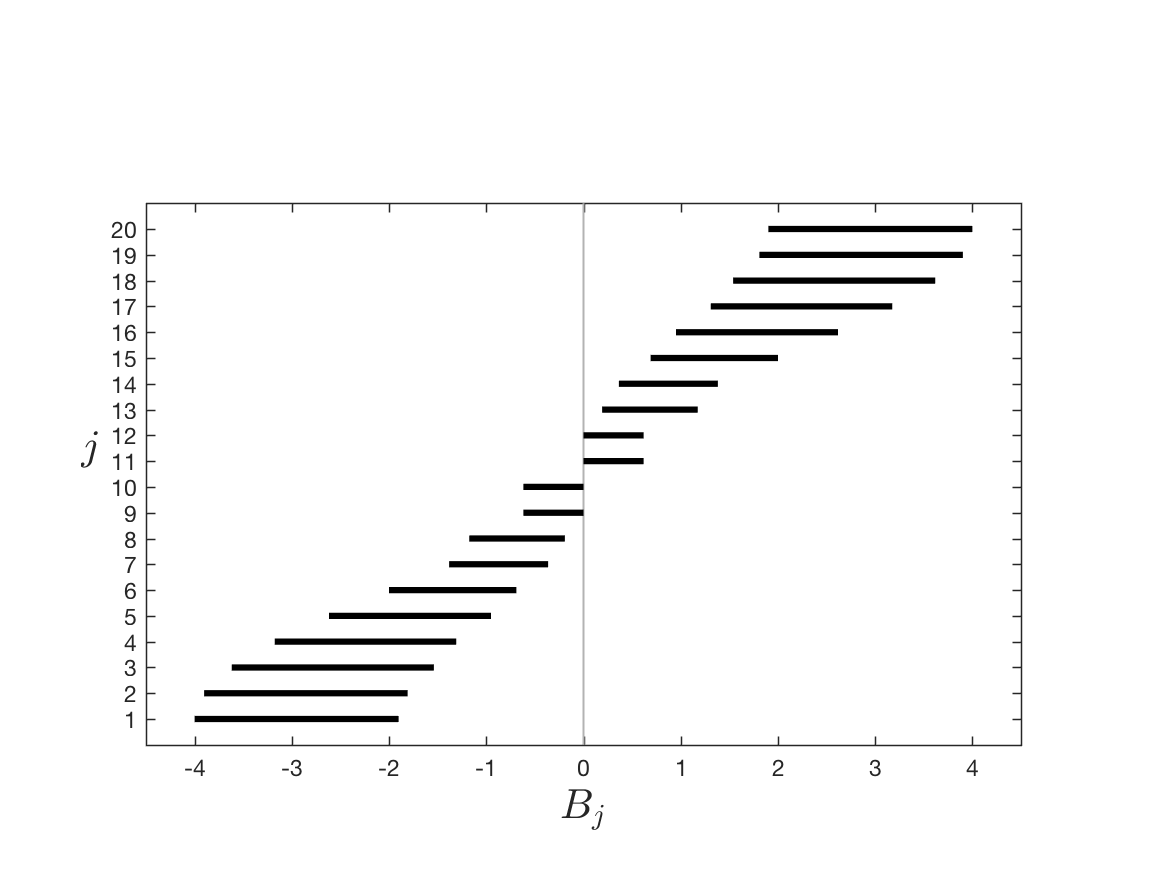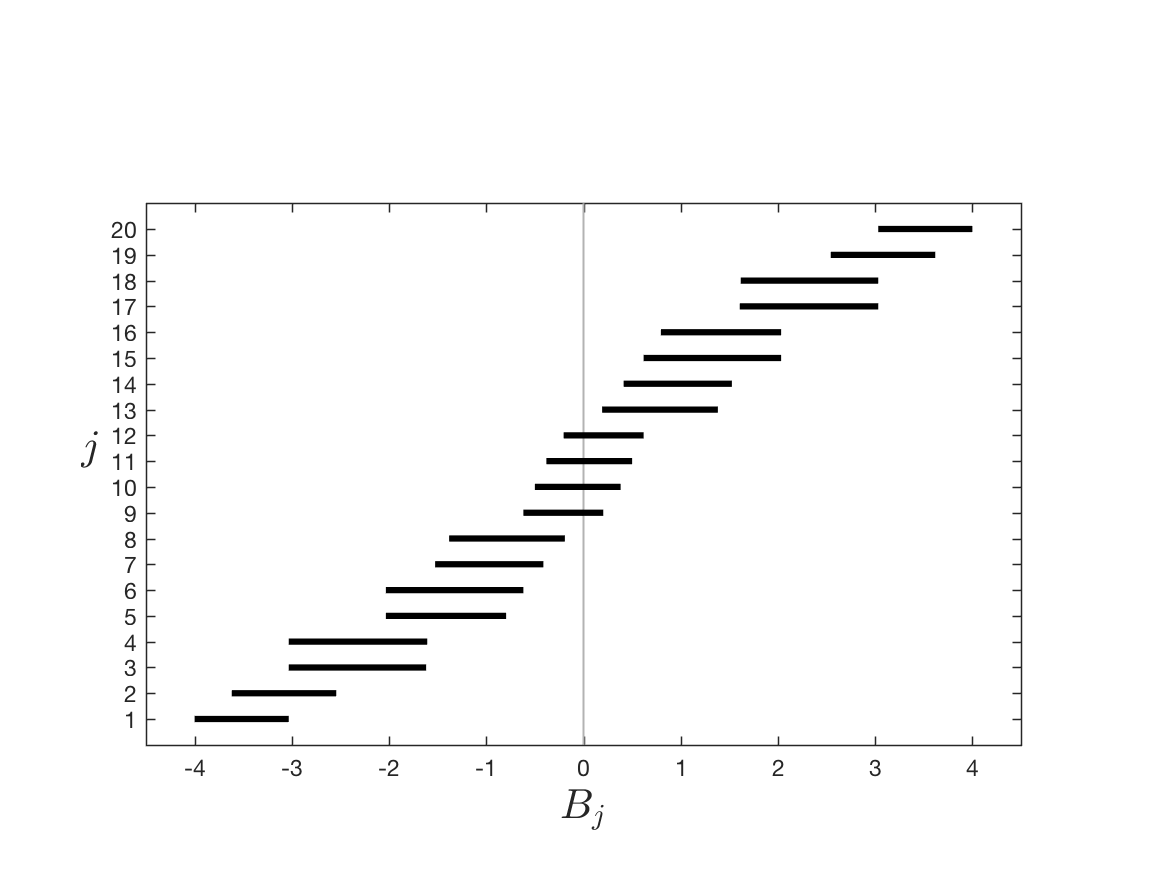The twenty spectral bands for the Laplacian with $p=2$, $q=10$ (left) and $p=5$, $q=4$ (right). A gap can only open at some value of $E\in(-4,4)$ that does not fall in the interior of any of these bands. We show that when $p$ and $q$ are both even, this is possible only at $E=0$. When either of $p$ or $q$ is odd, no gaps can open.

To investigate whether a gap can open at a given value $E$, we study the number of eigenvalues of $H_{\theta,\varphi}$ that fall strictly below $E$. For most values of $E$ this is easy to show; a set of $pq$ values require more careful atttention. The plots below show a few examples.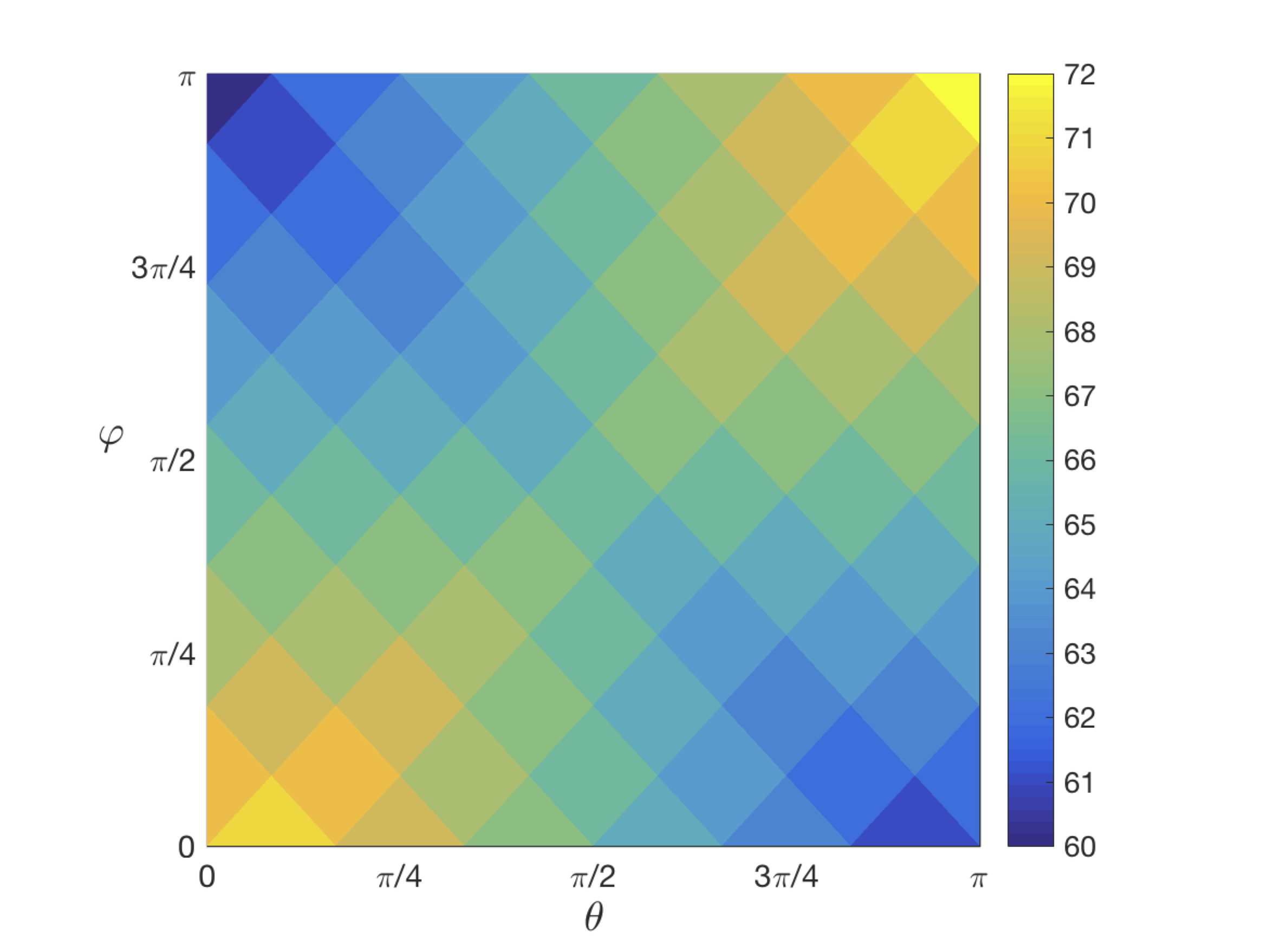Number of eigenvalues below $E=0$ for $p=11$ and $q=12$.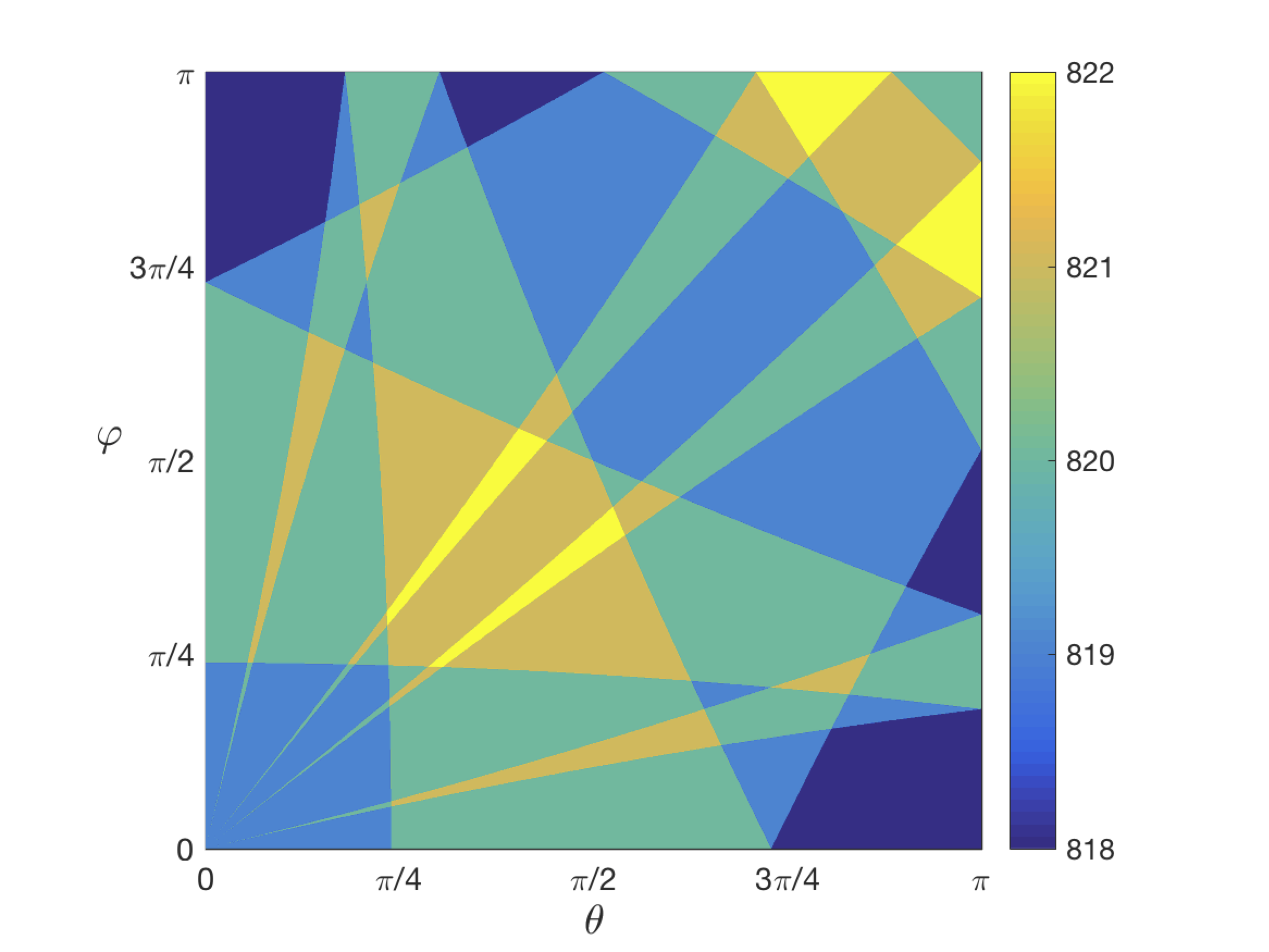Number of eigenvalues below $E=2\cos(\pi/3)+2\cos(\pi/15)$ for $p=q=30$.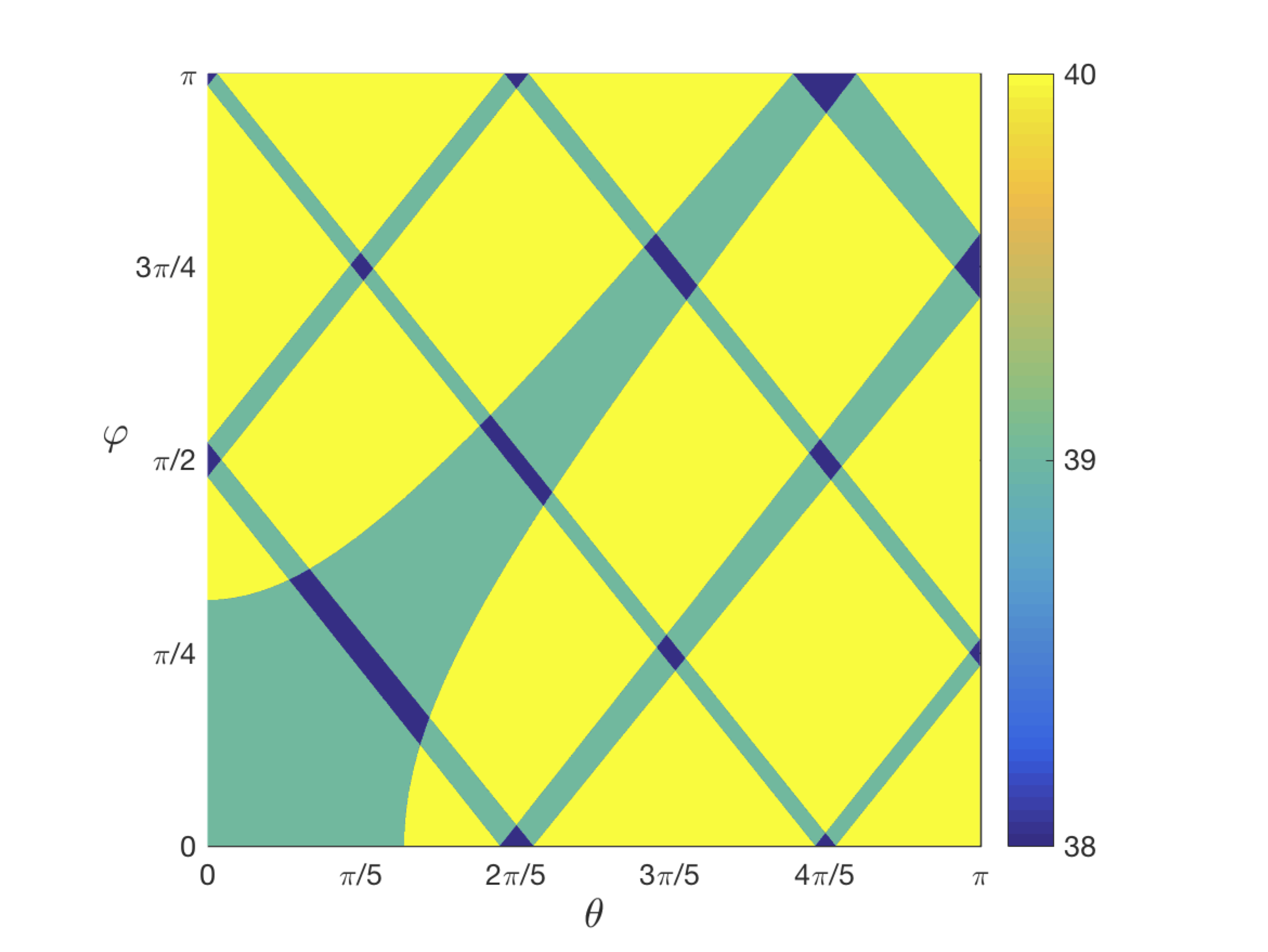Number of eigenvalues below $E=-0.01$ for $p=8$ and $q=10$.